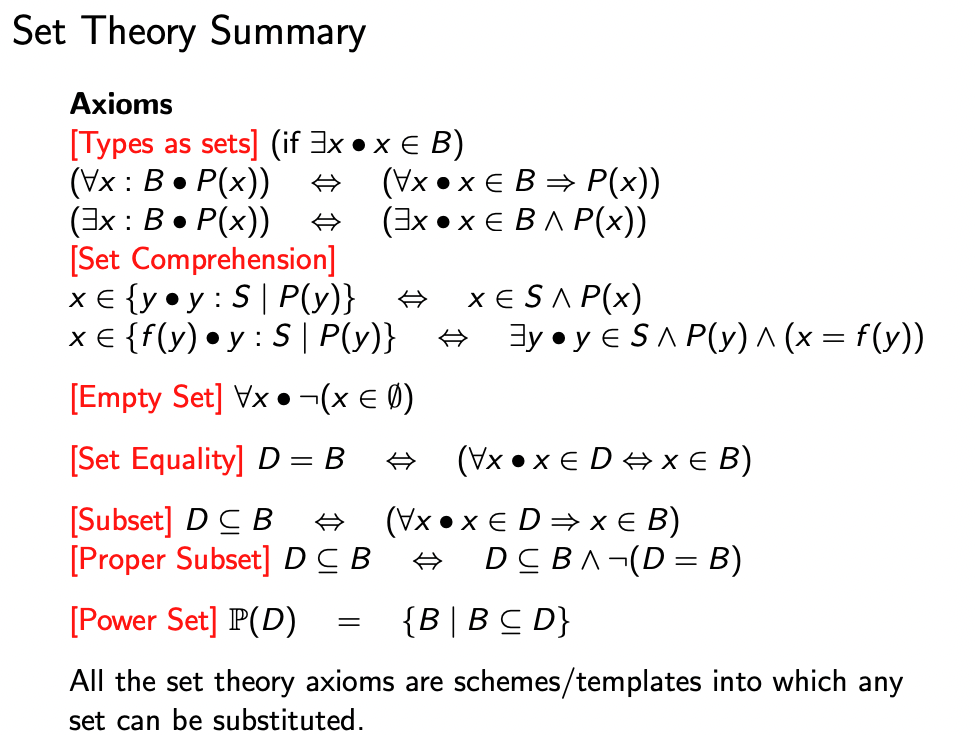# Set §

A set is a well-defined, unordered collection of distinct objects. Each object that appears in this collection is called an element (or member) of the set.

Examples (using set enumeration):

1. is a set that contains three elements.

### Empty Set §

The set contains no elements and is known as the empty set. We often use as the symbol.

Remark: Cardinality of : Number of elements in a finite set , denoted by Ex:

### Set-builder Notation (Set Comprehension) §

The idea is to define a set using a predicate; in particular, the set consists of all values that make the predicate true.

### Set Operations §

Union

The union of two sets and , written , is the set of all elements belonging to either set or set (or both). Symbolically we write

Intersection

The intersection of two sets and , written , is the set of all elements belonging to both set and set . Symbolically we write

Set-difference

The set-difference of two sets and , written (or ), is the set of all elements belonging to set but not . Symbolically we write

Complement

The complement of a set , written , is the set of all elements not in . Symbolically we write

### Prove Subset §

To prove that , prove the universally quantified implication:

### Prove Equal Subsets §

To prove that , we prove that and .

### SE212 §#### Axioms §

Types as sets: if

Set Comprehension

Empty Set

Universal Set The universal set consists of all the values of concern in any discussion (domain)

Set Equality

Subset

Proper Subset

Power Set The power set of a set is the set of all of its subsets. is the function that returns the power set of a set.

#### Other §

A singleton set is a set consisting only of one element.

#### Axioms for Set Functions §

Set Union Set Intersection

Two sets, and , are disjoint if their intersection is empty, i.e. . Serendipity -> Disjoint Set Union

Absolute Complement is the universal set.

Set Difference Note that for set difference, the order of operands MATTERS, i.e.

For example, let , and .

Cartesian product (Set Product)

Also see Binary Relation for the list of other properties.